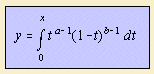# incomplete beta function

## gammaThe incomplete beta function Βx(a,b) is a variation on the beta function.

Sometimes the function is normalized to the regularized incomplete beta function I(x,a,b), defined as: Βx(a,b) / Β(a,b).The incomplete beta function and its inverse are used in statistics.
McKee (1993, 1995) et al. use the distribution in precipitation models.